## Eilenberg-Steenrod Axioms

A family of Functors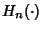from the Category of pairs of Topological Spaces and continuous maps, to the Category of Abelian Groups and group homomorphisms satisfies the Eilenberg-Steenrod axioms if the following conditions hold.

1. Long Exact Sequence of a Pair Axiom. For every pair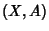, there is a natural long exact sequence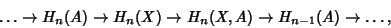(1)

where the Map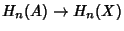is induced by the Inclusion Map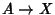and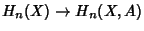is induced by the Inclusion Map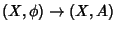. The Map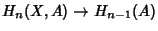is called the Boundary Map.

2. Homotopy Axiom. If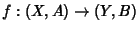is homotopic to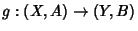, then their Induced Maps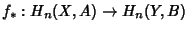and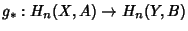are the same.

3. Excision Axiom. If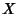is a Space with Subspacesand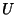such that the Closure ofis contained in the interior of, then the Inclusion Map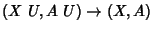induces an isomorphism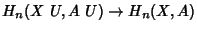.

4. Dimension Axiom. Letbe a single point space.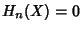unless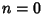, in which case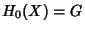where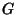are some Groups. The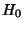are called the Coefficients of the Homology theory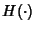.

These are the axioms for a generalized homology theory. For a cohomology theory, instead of requiring thatbe a Functor, it is required to be a co-functor (meaning the Induced Map points in the opposite direction). With that modification, the axioms are essentially the same (except that all the induced maps point backwards).